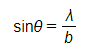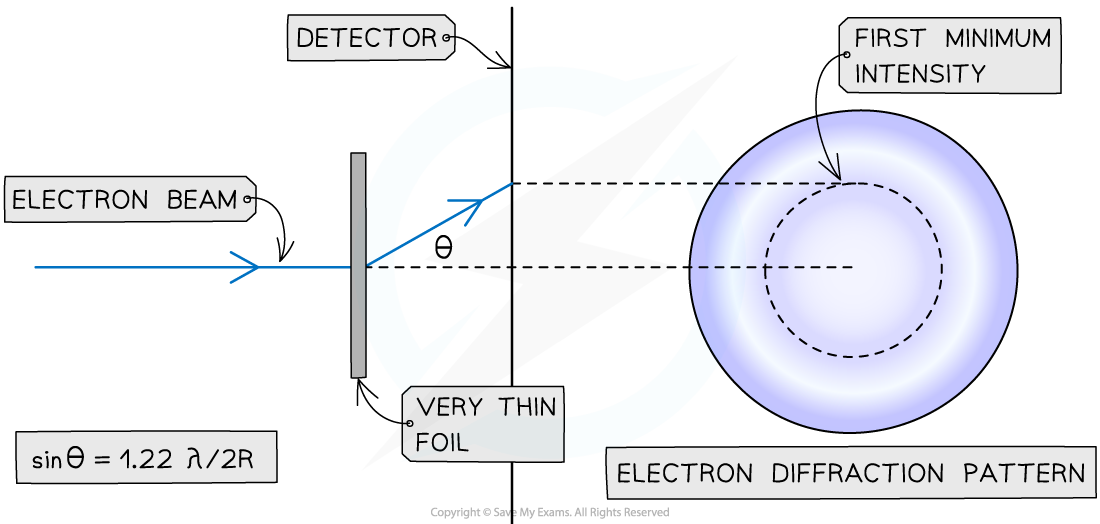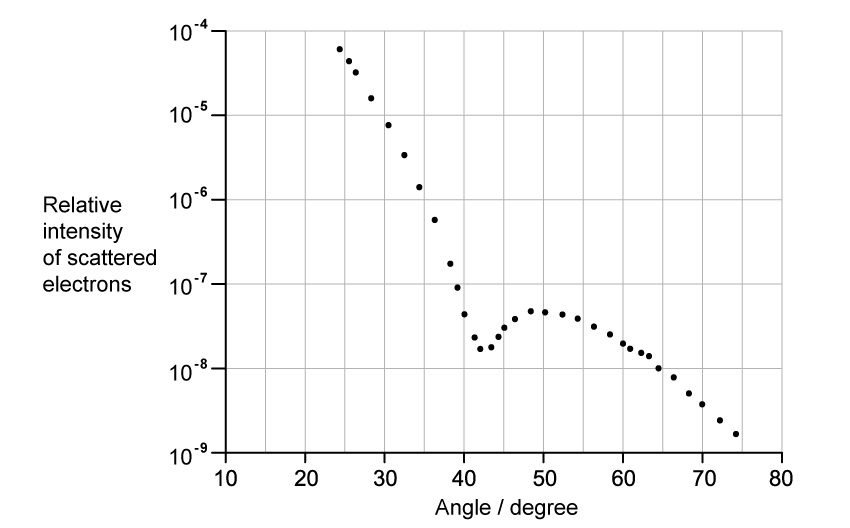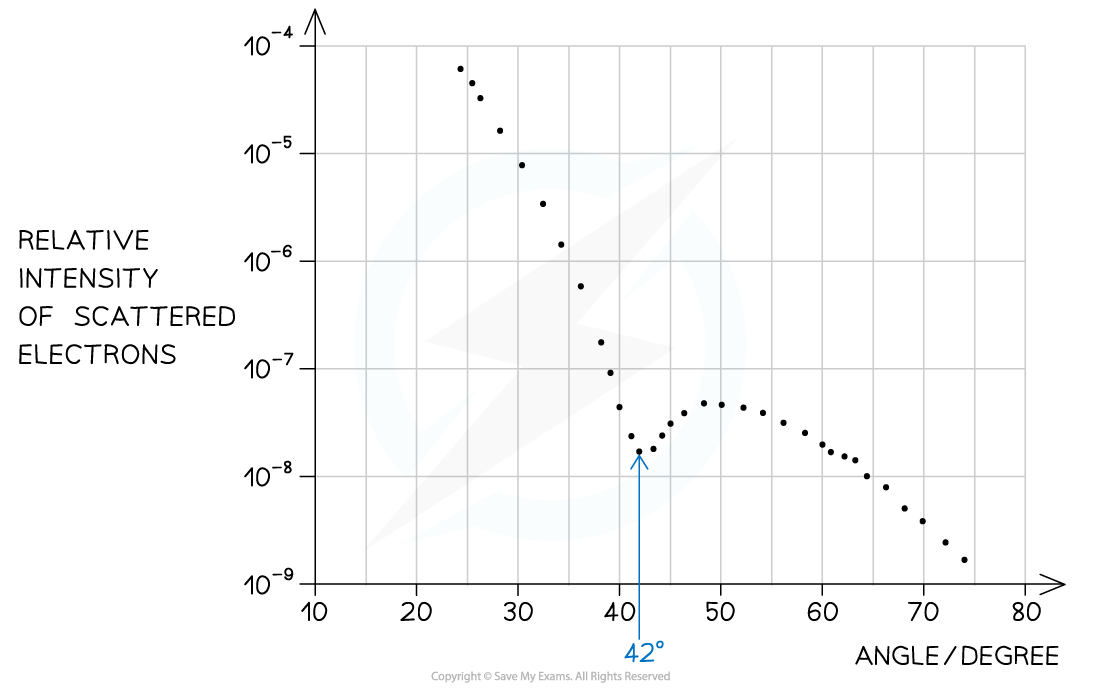# IB DP Physics: HL复习笔记12.2.2 Nuclear Scattering

### Nuclear Scattering

• Electrons accelerated to close to the speed of light have wave-like properties such as the ability to diffract and have a  de Broglie wavelength equal to:• Where:
• h = Planck's constant
• m = mass of an electron (kg)
• v = speed of the electrons (m s−1)
• When beams of neutrons or electrons are directed at a nucleus they will diffract around it
• The pattern formed by this diffraction has a predictable minimum which forms at an angle θ to the original direction according to the equation• The diffraction pattern forms a central bright spot with dimmer concentric circles around it
• From this pattern, a graph of intensity against diffraction angle can be used to find the diffraction angle of the first minimum
• The graph of intensity against angle obtained through electron diffraction is as follows:The first minimum of the intensity-angle graph can be used to determine nuclear radius

• Using this, the size of the atomic nucleus, R, can be determined from:• Where:
• θ = angle of the first minimum (degrees)
• λ = de Broglie wavelength (m)
• R = radius of the nucleus (m)Geometry of electron diffraction

#### Worked Example

The graph shows how the relative intensity of the scattered electrons varies with angle due to diffraction by the oxygen-16 nuclei. The angle is measured from the original direction of the beam.The de Broglie wavelength λ of each electron in the beam is 3.35 × 10−15 m.

Calculate the radius of an oxygen-16 nucleus using information from the graph.

Step 1: Identify the first minimum from the graph• Angle of first minimum, θ = 42°

Step 2: Write out the equation relating the angle, wavelength, and nuclear radiusStep 3: Calculate the nuclear radius, R